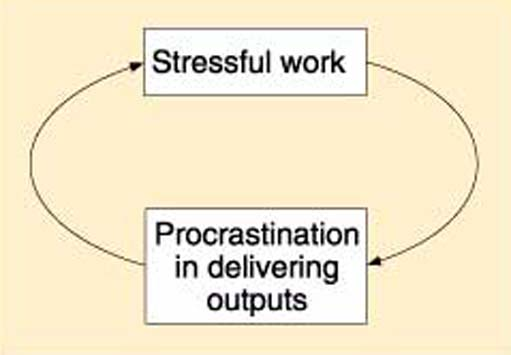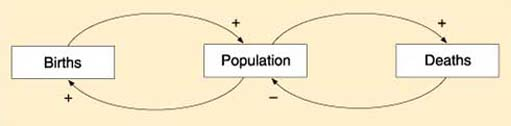Understanding the environment: Flows and feedback

Start this free course now. Just create an account and sign in. Enrol and complete the course for a free statement of participation or digital badge if available.

Free course

# Reading 4.6: Representing feedback through sign graph diagrams

Reading 2.5 illustrated how difficult it is to depict relational information using verbal communication, and the need for other forms of representation that move away from the linear, ‘drilling down’ reductionist approach.

This reading introduces you to the most popular diagramming technique used to represent systems, made famous by Peter Senge’s (Senge, 1994) best-selling book, The Fifth Discipline, where sign graph diagrams are used to represent systems archetypes (classic behavioural feedback traps people get themselves into). (Note that the use of the term ‘sign graph’ is actually unique to the Open University. All other literature refers to this diagramming technique as ‘causal loop diagrams’.) The basic building blocks of sign graph diagrams are phrase couplets joined by an arrow in order to represent causal relationships. For example:

• food intake → weight gain;
• births → population growth;
• deforestation → reduction in rainfall;
• stressful work → procrastination in delivering outputs.

The phrase usually describes an activity or process (e.g. weight gain) happening to an object or one of its attributes. People do not usually bother to name the object in the phrase. In the weight gain example, it is referring to a human individual. The arrow signals that the change in the left-hand side object, or one of its attributes, will result in a change in the right-hand side object, or one of its attributes. The arrow is a symbolic representation of terms such as ‘causes’, ‘effects‘ or ‘influences’. The left-hand side object is sometimes referred to as the ‘explanatory variable’ and the right-hand side object as the ‘response variable’.

Sometimes, + and - signs or ‘S’ (representing the word ‘same’) and ‘O’ (representing the word ‘opposite’) are placed next to the arrow to indicate whether the change in the response variable is in the same direction as the explanatory variable (+ sign or ‘S’ symbol) or in the opposite direction (- sign or ‘O’ symbol).

Sign graph diagrams are excellent at communicating feedback relationships (see Figure 4.5). In some representations, the coupled variables are both explanatory and in response.Figure 4.5 Communicating feedback relationships through a sign graph diagram

In order to maintain some clarity in the diagram and not make it end up looking like a bowl of spaghetti, it is useful to have the principal feedback loop at the centre of the diagram and limits to the number of causal links coming in. Miller’s magic number seven, plus or minus two (as introduced in Reading 1.7), is a useful guideline in deciding how many variables to include. But this is only a guideline for the final diagram. The actual drawing would benefit from various drafts, with each emphasising analysis (breaking down relationships, increasingly detailed objects) and synthesis (combining objects and attributes to better reflect the purpose of the diagramming activity). One could visualise this as alternating phases of sweeping in relevant detail, and then sweeping out irrelevant information.

Although sign graph diagrams are relatively easy to develop, they do occasionally result in ‘logical’ pitfalls for example, when using the simple population dynamics model (see Figure 4.6).Figure 4.6 Pitfalls in sign graph diagramming

If we assume that the death rate is less than the birth rate, the positive feedback loop apparent in the diagram would imply that an increase in births would result in an increase in population. So far, that is pretty logically consistent. But, if we look at the diagram again and assume a drop in birth rates, the diagram would imply a drop in population. That is clearly not the case as long as death rates are lower than birth rates.

The next reading introduces you to system dynamics diagrams. This technique requires a little bit more effort than sign graph diagrams, but avoids the logical pitfalls outlined above.

T214_5